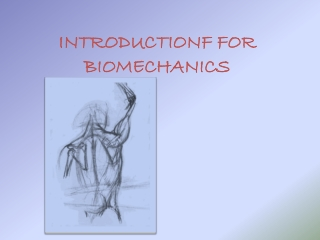DownloadDownload PresentationINTRODUCTIONF FOR BIOMECHANICS

# INTRODUCTIONF FOR BIOMECHANICS

Download Presentation## INTRODUCTIONF FOR BIOMECHANICS

- - - - - - - - - - - - - - - - - - - - - - - - - - - E N D - - - - - - - - - - - - - - - - - - - - - - - - - - -
##### Presentation Transcript

1. INTRODUCTIONF FOR BIOMECHANICS

2. When we study any part of knowledge we ask ourselves these questions • What Is the application of mechanical principles on the living organisms. • Why Via this science we able to solve many medical problems that face the patients • How Using it on designing exercise programs, orthotics and prosthesis, application of a technique etc… even when we give an advice to a patient.

3. Course Contents • Forces • Centre of Gravity (COG). • Stability and Equilibrium. • Force Systems. • Simple Body Mechanics. • Motion and Newton’s laws.

4. Biomechanics of bone. • Behaviour of bone under stress. • Stress strain curve. • Possible fracture patterns. • Various loading modes.

5. Biomechanics of fracture fixation. • Soft tissue biomechanics(muscle and cartilage) • Regional biomechanics: hip, knee, shoulder, spine. • normal Gait. • Pathomechanics of Gait. • Mechanics of Posture.

6. Kinesiology &Biomechanics • Kinesiology is the scientific study of human motion. (kinesis and ology) • Biomechanics Is the application of mechanical principles on the living organisms.

7. Forces • Definition: Physical quantity that tends to change state or shape of an object ( state means the speed or equilibrium). So force can generate, stop, or modify motion. It’s unit is Newton (N).

8. Force is a vector quantity That because it has a magnitude and direction. Represented graphically by an arrow(vector) has four characteristics: 1-Magnitude  length 2-Direction  head 3-point of application tail 4-line of application angle with the horizontal (angle of pull).

9. A Vector representing a force (2) (1) (4) (3)

10. Gravitational force The force that attract any object to the Earth. The force by the gravity on an object is equal to its weight . W = M x (g) (in Newton) (in kg) Where g=9.8m/s or =10 N/kg 2

11. Magnitude= the weight of the body. • Direction= downward. • Point of application= the center of gravity. • Line of application= 90 angle (perpendicular to the ground) (COG is an imaginary point that the body weight can be assumed to be concentrated and equally distributed.)

12. Ground Reaction Force • Magnitude = gravity. • Direction opposite to gravity. • Line of application= gravity. • Point of application= point of contact to the ground.

13. Frictional force It’s the resistance force generated when two surfaces pressed and slide on each others. • Magnitude= the pressure and coefficient of friction (µ) roughness. • Direction=lateral. • Point of application= the surface of contact. • Line of application= parallel to the surface

14. pressure Definition: It is the total force applied per unit area. P=F/A Measured in (N/m2 ) or pascal

15. Resistance force that tends to reduce or stop a moving object As air or water resistance, it depends mostly on the surface area

16. Center Of Gravity(COG) Definition:(center of mass) COG is an imaginary point that the body weight can be assumed to be concentrated and equally distributed, around which body can rotate freely in all direction. At which summation of all moments equal zero

17. Location of COG • Depends on the body’s shape and position. • In objects possess a symmetrical shape and it’s mass are equally distributed it is located exactly in the center. • For asymmetrical irregular bodies the COG will be nearer the larger and heavier end. • In normal standing adult person its located anterior to the second sacral vertebra.

18. Factors affect the location of COG in the human body • Age • In newborn: above the umbilicus. • At two years: at level of umbilicus. • At five years: below the level of the umbilicus. • In adults: anterior to the 2nd sacral vertebra.

19. 2-Sex The COG in males is higher than in females Because of The different mass distribution

20. 3- position of any part in relation to the others COG is always shifts to the heavy mass e.g. flexion and extension running.

21. 4-Addition and subtraction of weight • By carrying a weight • Pregnancy • amputation